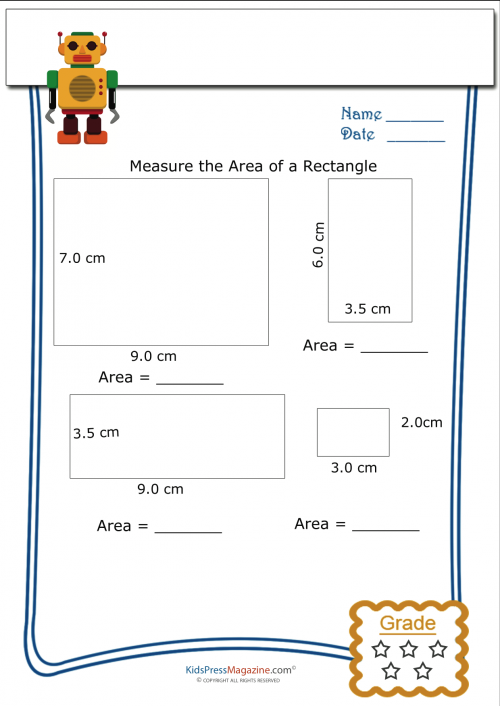# Measuring Area Worksheet – Rectangle 5

Learning algebra is a lot easier when you have visuals. That is why geometry is such a great aid to children learning basic algebra. The formula A = HW is algebraic in nature and solves the geometry question of “what is the area of a rectangle?” (Area = Height x Width)